Courses
Courses for Kids
Free study material
Free LIVE classes
More

# JEE Chapter - States of MatterLIVE
Join Vedantu’s FREE Mastercalss

## Introduction to States of Matter

The bulk properties of matter, i.e the properties of matter that we are familiar with, are represented by the characteristics of chemical systems with which we are familiar. Properties are associated with a large number of items like a collection of atoms, ions, or molecules. A liquid's molecules do not boil, but the bulk does. Water molecules in a collection have wetting properties; Molecules do not wet on their own. Ice is a form of water. It can exist as a solid, as a liquid, or in a gaseous state. Water vapour or steam in a gaseous state. Ice, water, and steam can have very different properties. In the chemical composition of water in all three states, H2O continues to be the same.

## JEE Main Chemistry Chapter-wise Solutions 2022-23

### Important Topics of States of matter

• Intermolecular Forces

• Thermal energy

• The gaseous state

• The Gas Laws

• Ideal gas equation

• Kinetic energy and molecular speeds

• Kinetic molecular theory of gases

• Behaviour of real gases

• Liquefaction of gases

• Liquid state

### 1. Intermolecular Forces

The forces of attraction and repulsion between interacting particles are known as intermolecular forces (atoms and molecules). This phrase is used to describe does not take into account the electrostatic forces that exist between the two ions with opposing charges and the forces that hold a molecule's atoms together.

### (a) Dispersion Forces or London Forces

• Because their electronic charge cloud is evenly distributed, atoms and nonpolar molecules are electrically symmetrical and have no dipole moment. Even with such atoms and molecules, however, a dipole can form for a brief period of time.

• The interaction energy is inversely proportional to the sixth power of the distance between two interacting particles, and these forces are always attractive.

### (b) Dipole-Dipole Forces

• Between molecules with permanent dipoles, dipole-dipole forces act. The dipoles' ends have "partial charges," which are represented by the Greek character delta .

• Partial charges are always less than the unit electronic charge. The polar molecules interact with their neighbours.

### (c) Dipole–Induced Dipole Forces

• This form of attraction exists between polar molecules that have a persistent dipole and molecules that do not have a permanent dipole.

• By deforming the electronic cloud of the electrically neutral molecule, the permanent dipole of the polar molecule produces a dipole on the electrically neutral molecule.

### (d) Hydrogen Bond

• This bond is found in compounds that include extremely polar N–H, O–H, or H–F bonds. Although hydrogen bonding is thought to be limited to the elements N, O, and F, species like Cl can also participate.

• The energy of a hydrogen bond can range from 10 to 100 kJ mol–1. Because hydrogen bonds have such a large quantity of energy, they are a potent factor in influencing the structure and characteristics of many substances, such as proteins and nucleic acids.

• The coulombic interaction between the lone-pair electrons of one molecule's electronegative atom and the hydrogen atom of another molecule determines the strength of the hydrogen bond.

### 2. Thermal Energy

• Thermal energy is a body's energy derived from the motion of its atoms or molecules. It is directly proportional to the substance's temperature. It is the measure of average kinetic energy of matter particles and is consequently responsible for particle movement. Thermal motion is the name given to this type of particle movement.

### 3. Intermolecular Forces vs Thermal Interactions

• When molecular interactions are weak, molecules do not stick together to form a liquid or solid until the temperature is lowered to minimise thermal energy. Although molecules get very close to one another and intermolecular tensions are at their highest, -gases do not liquefy solely due to compression.

• When the thermal energy of molecules is reduced by lowering the temperature, the gases become easier to liquefy.

### 4. The Gaseous State

We spend our entire lives immersed in the ocean of air, which is made up of a variety of gases. We spend our lives in the troposphere, the lowermost layer of the atmosphere that is held to the earth's surface by gravitational force.

The physical properties that define the gaseous state are as follows.

• Gases can be compressed quite easily.

• Gases apply pressure in all directions equally.

• The density of gases is substantially lower than that of solids and liquids.

• Gases do not have a definite volume or shape.

• Gases mix uniformly and fully in all amounts without any mechanical assistance, assuming the container's capacity and shape.

### (a) Boyle’s Law (Pressure-Volume Relationship)

Robert Boyle came to the conclusion that the pressure of a fixed amount (i.e., number of moles n) of gas varies inversely with its volume at constant temperature based on his experiments. Boyle's law is the name for this. It can be written as -

P1/V1=P2/V2 at the constant temperature.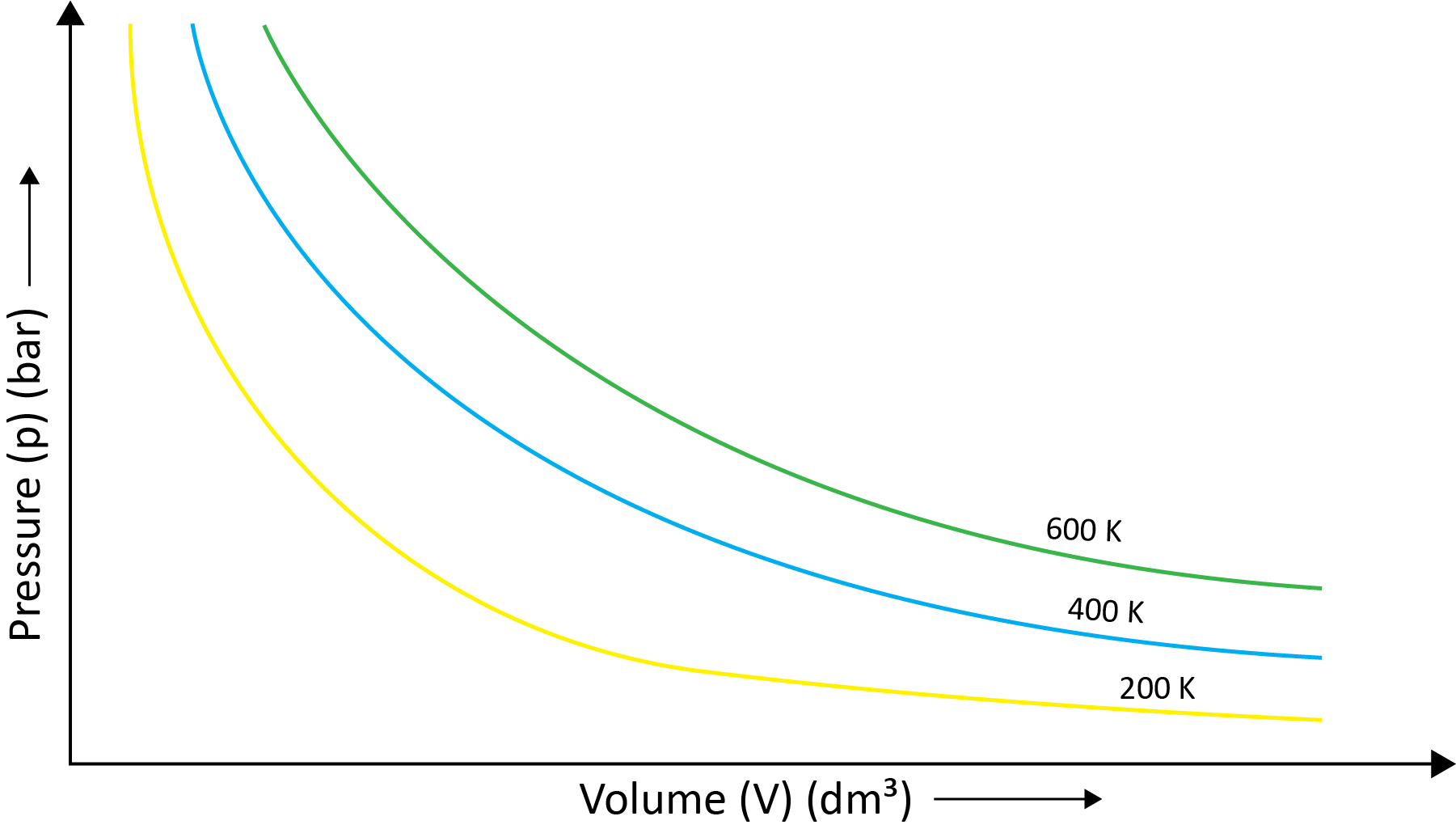### (b) Charles’ Law (Temperature - Volume Relationship)

Charles and Gay Lussac individually conducted various gas experiments in order to advance hot air balloon technology. Their research revealed that the volume of a gas increases with increasing temperature and decreases with cooling for a fixed mass of a gas at constant pressure. The mathematical expression can be given as at the constant volume and pressure-

V1/T1=V2/T2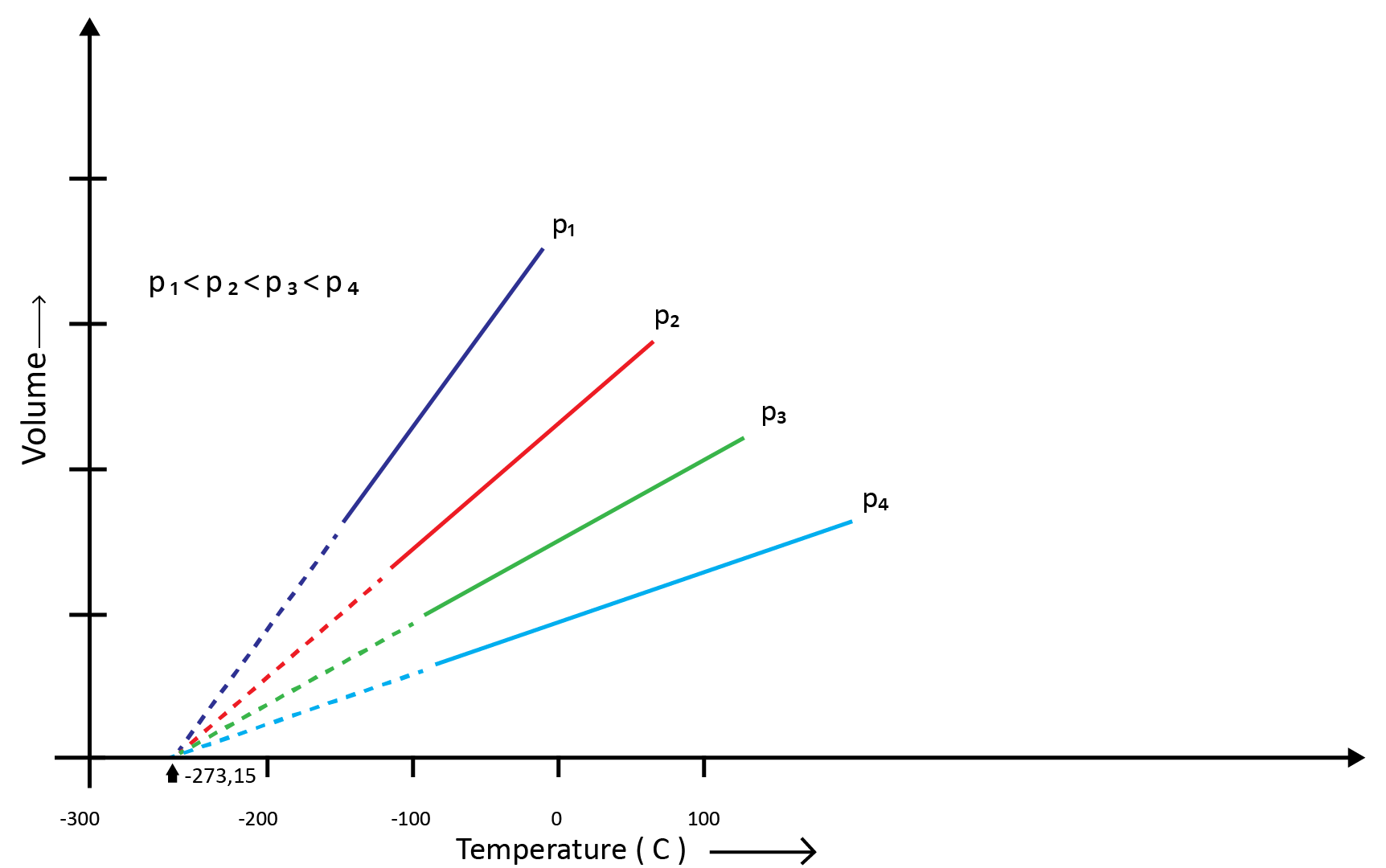### (c) Gay Lussac’s Law (Pressure-Temperature Relationship)

Joseph Gay Lussac established the mathematical link between pressure and temperature, which is known as Gay Lussac's law. It claims that the pressure of a fixed amount of gas varies directly with the temperature at constant volume. Mathematically,

P1/T1=P2/T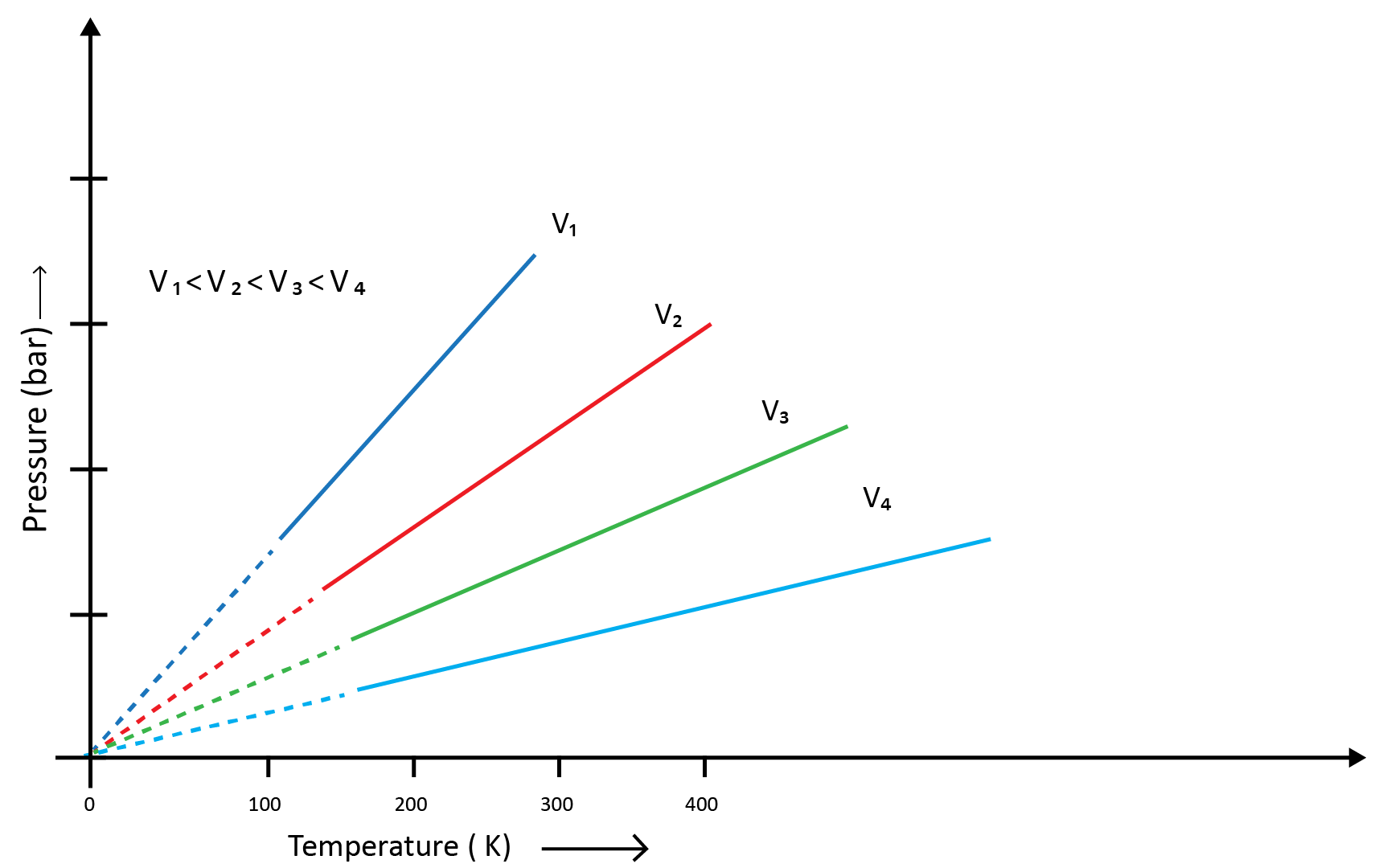Boyle's and Charles' laws can be used to draw this relationship. The above  graph of pressure vs. temperature (Kelvin) at constant molar volume. Isochore refers to each line in the above graph.

### (d) Avogadro Law (Volume - Amount Relationship)

It says that under the same temperature and pressure, identical volumes of all gases contain the same number of molecules. This means that the volume of a gas is determined by the number of molecules in the gas, or the amount of gas, as long as the temperature and pressure remain constant. Mathematically-

V1/n1=V2/n2

### The three laws ( Boyle's law, Charles law, Avogadro's law) that we've learned so far can be merged into a single equation known as the ideal gas equation-

pV=nRT

R is called gas constant. It is the same for all gases. Hence,  it is also called Universal Gas Constant.

### (a) Density and Molar Mass of a Gaseous Substance

The relation is written below-

d/M = P/RT

### (b) Dalton’s Law of Partial Pressures

John Dalton drafted the legislation in 1801. It asserts that the overall pressure exerted by a mixture of non-reactive gases is equal to the sum of individual gas partial pressure.

### 7.  Kinetic Theory and Molecular Speeds

• Gas molecules are constantly moving. They crash with each other and the container's walls as they move. As a result, their speed changes and energy is redistributed. As a result, the speed and energy of all the gas molecules at any given time are not the same.

• The true distribution of molecular speeds in a gas is dependent on temperature and molecule mass, as demonstrated by Maxwell and Boltzmann.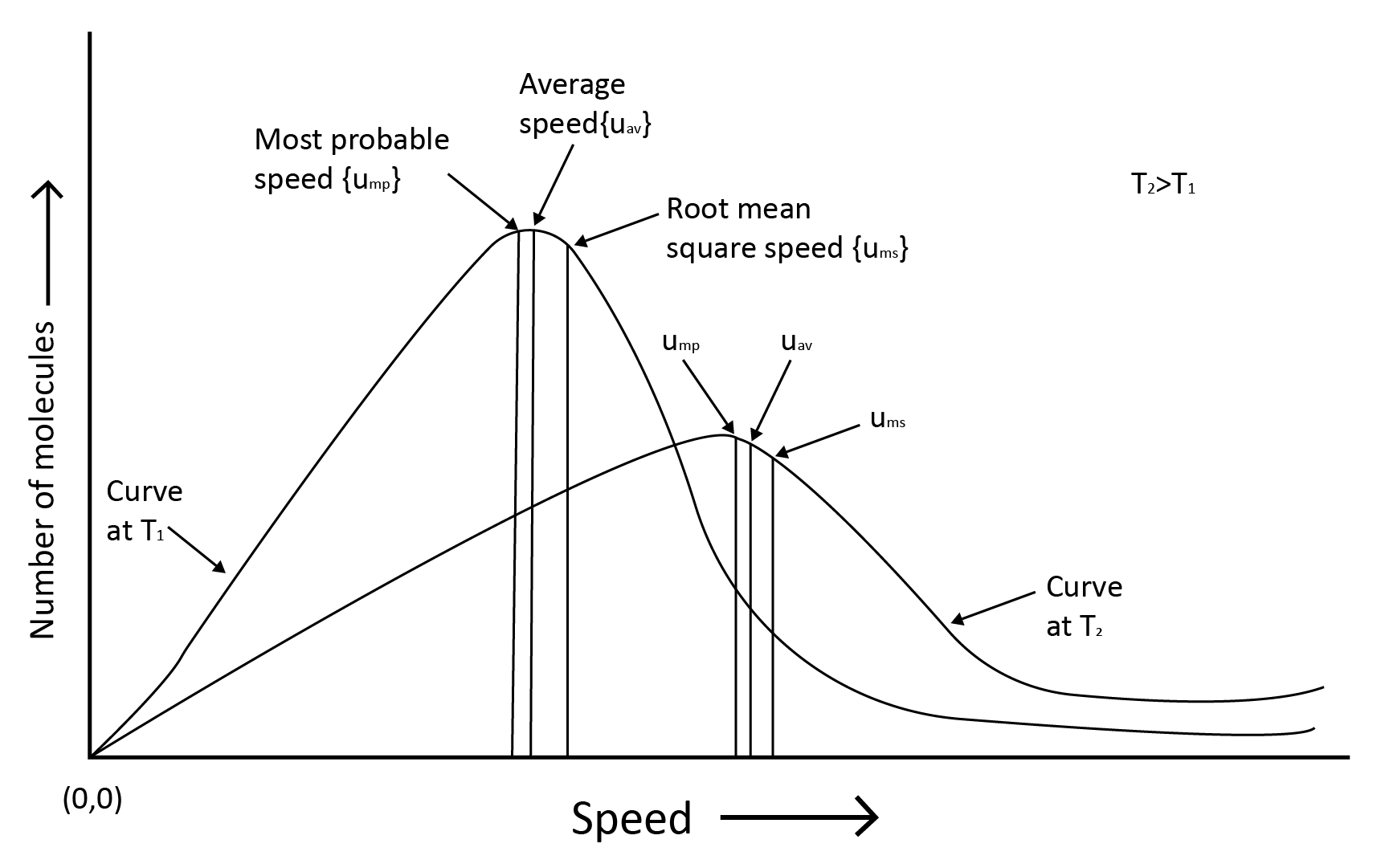• Root mean square speed, average speed and the most probable speed have the relationship:  urms > uav > ump

### 8. Kinetic Theory of Gases

The postulates are given below-

1. Gases include a huge number of microscopic particles.

2. A gas exerts pressure on an object.

3. There is no kinetic energy loss.

4. Gas molecules are attracted to one another.

5. The molecule's kinetic energy is proportional to its absolute temperature.

6. The gaseous molecule's actual volume is relatively small.

7. Gaseous molecules are always moving.

8. Gravity has a greater impact on the movement of gaseous molecules.

### 9. Behaviour of Real Gases

When a pressure v/s volume plot is drawn, deviation from optimal behaviour is also visible. The experimental pressure vs volume plot (actual gas) and the theoretical pressure v/s volume plot (ideal gas) should be identical. This graph is shown below. The measured volume is clearly greater than the calculated amount at very high pressure.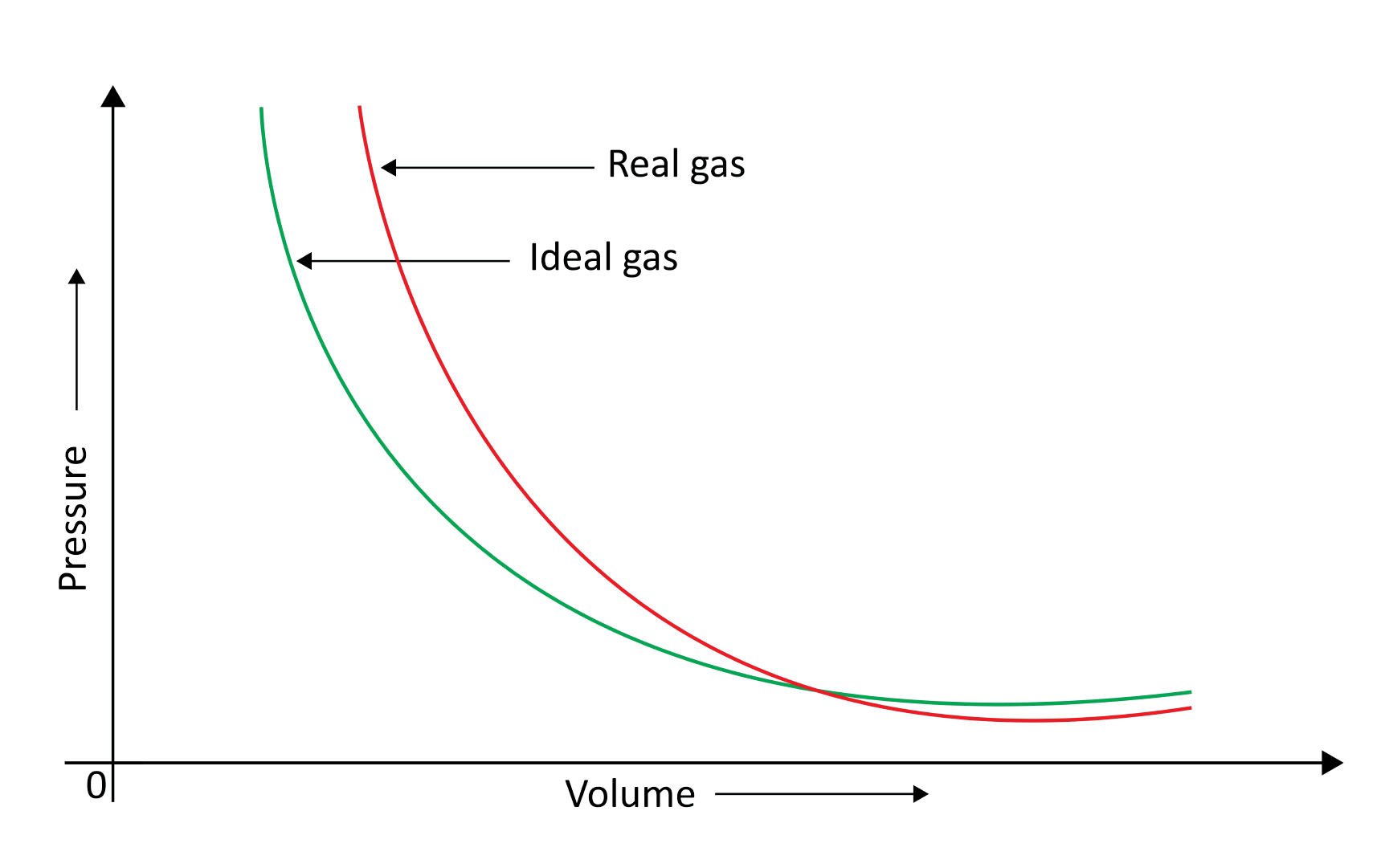Because molecules interact with one another, real gases deviate from the ideal gas law.

At high pressures, gas molecules are extremely near to one another. Molecular interactions begin to take place. Molecules do not strike the container walls with full power at high pressure because they are pulled back by other molecules due to molecular attraction forces.

• This has an impact on the pressure that molecules exert on the container's walls. As a result, the pressure exerted by the gas is lower than that of the ideal gas.

• The compressibility factor Z, which is the ratio of product pV and nRT, can be used to calculate the departure from ideal behaviour.

Z =pV/nRT is a mathematical formula.

Because pV = nRT, Z = 1 for an ideal gas at all temperatures and pressures. The value of Z deviates from unity for gases that deviate from ideality. All of the gases presented have Z =1 and behave like ideal gases at very low pressures. At high pressure, all gases have a Z>1. Compressing these is more challenging. Most gases have Z<1 at moderate pressures.

### 10. Liquefaction of Gases

Andrews noted that isotherms at high temperatures resemble those of an ideal gas and that the gas cannot be liquefied even at very high pressure. The shape of the curve varies as the temperature drops, and the data shows a significant divergence from optimal behaviour. Carbon dioxide remains a gas up to 73-atmosphere pressure at 30.98°C (point E.) Liquid carbon dioxide occurs for the first time at 73 atmosphere pressure. The temperature of 30.98°C is known as the carbon dioxide critical temperature. This is the temperature at which liquid carbon dioxide can be found. It is gas above this temperature. Critical volume and critical pressure are terms used to describe the volume of one mole of a gas at a critical temperature.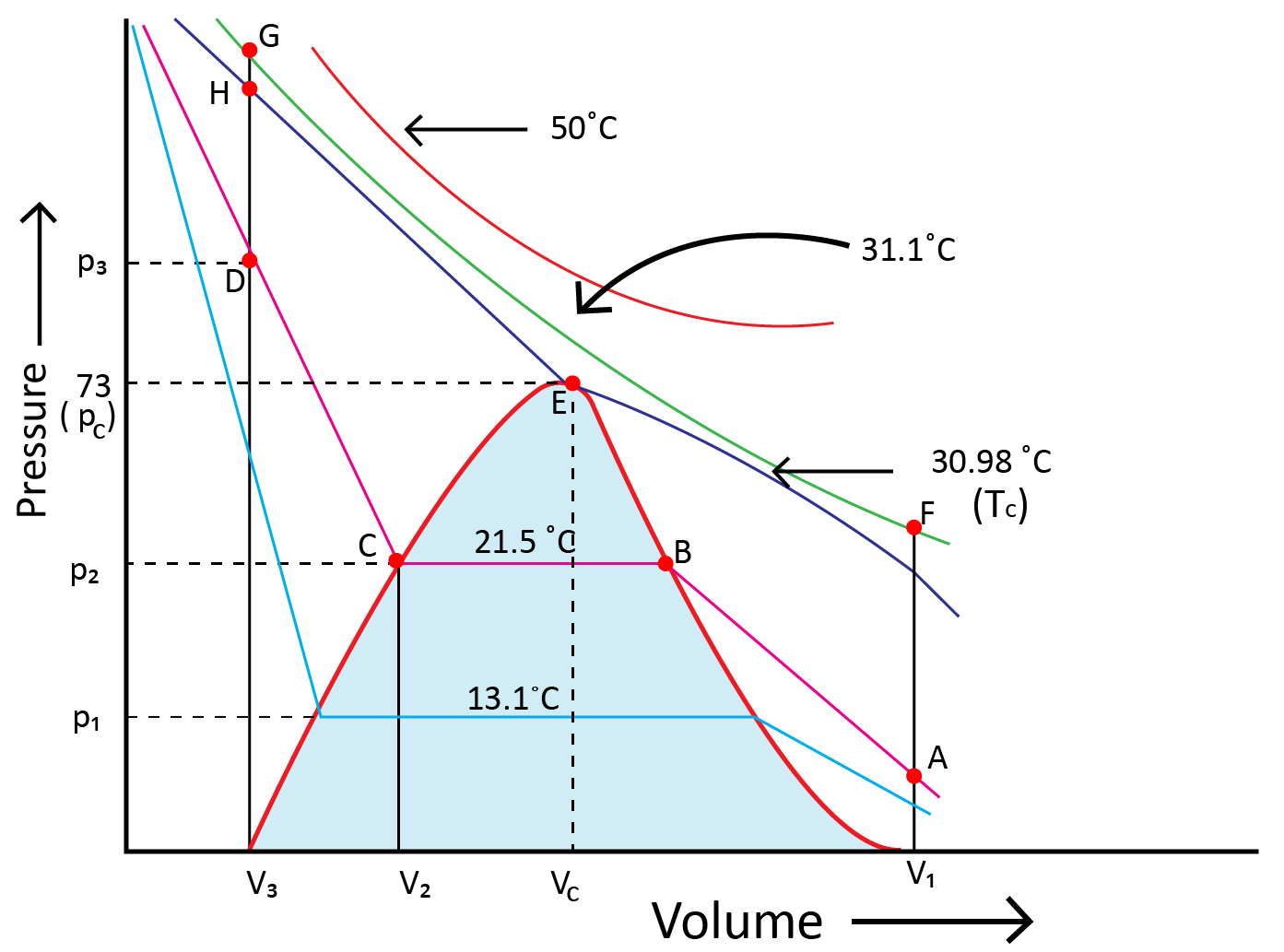### 11. Liquid State

In the liquid state, intermolecular forces are stronger than in the gaseous state. Liquid molecules are so close together that there is very little empty space between them, thus liquids are denser than gases under typical conditions. Liquid molecules are held together by intermolecular forces that are attractive. Because molecules do not split from one another, liquids have a defined volume.

### (a) Vapour Pressure

If a partially filled evacuated container is a liquid in which a portion of the liquid evaporates in order to replenish the container's remaining volume with vapour. The liquid evaporates at first, and pressure is placed on the walls of a vessel by vapours The pressure inside the container (vapour pressure) rises. After that, It eventually settles into a steady state, and a state of equilibrium is established between the liquid and solid phases  and phase of vaporisation At this point, the vapour pressure is high. Vapour pressure at this stage is called equilibrium vapour pressure or saturated vapour pressure.

### (b) Surface Tension

• Surface tension is the tendency of liquid surfaces to shrink to the smallest possible surface area while they are at rest.

• In the bulk of a liquid, a molecule is subjected to equal intermolecular forces from all sides. As a result, the molecule is not subjected to any net force. However, the net attractive force for a molecule on a liquid's surface is towards the liquid's interior.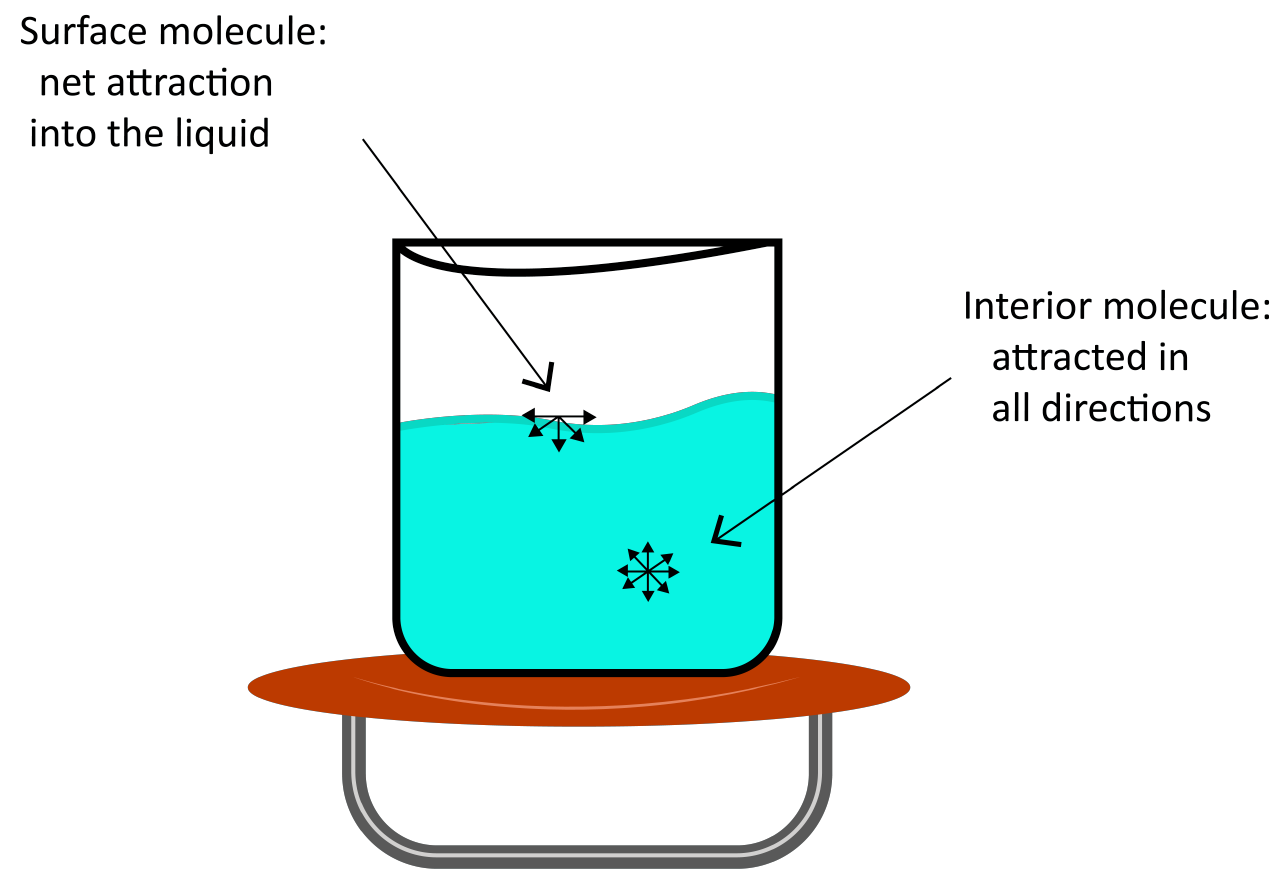### (c) Viscosity

• It is one of the distinguishing characteristics of liquids. Internal friction between layers of fluid as they slip past one another while liquid flows cause viscosity, which is a measure of flow resistance. Strong intermolecular interactions hold molecules together and prevent layers from moving past one another.

• The viscosity of a liquid determines how slowly it moves. The van der Waals and hydrogen bonding forces are strong enough to generate significant viscosity. Glass is a viscous liquid with a high viscosity.

• It has such a high viscosity that many of its properties are similar to those of solids.

• Because at high temperatures, molecules have enormous kinetic energy and can overcome intermolecular interactions to glide past one another between layers, the viscosity of liquids lowers.

### Solved Examples from the Chapter

Example 1: Calculate the compressibility factor for CO2, if one mole of it occupies 0.4 litre at 300K and 40 atm. Comment on the result.

Solution: Compressibility factor (Z) = PV / nRT

Z = 40 × 0.4 / 1 × 0.0821 × 300 = 0.65

As, the Z value is lesser than 1 and thus, nRT > PV. In order to have Z = 1, volume of CO2 must have been more at the same P and T or CO2 is more compressible than ideal gas.

Key point to remember:The compressibility factor Z, which is the ratio of product  of pV and nRT, can be used to calculate the departure from ideal behaviour.

Example 2: How large a balloon can you fill with 4g of He gas at 22°C and 720 mm of Hg?

Solution: Given, P = 720 / 760 atm, T = 295K, w = 4g  and M = 4 for He

PV = w / M RT   = 720 / 760 × V = 4/4 × 0.0821 × 295

Therefore, V = 25.565 litre.

Key point to remember: Ideal gas equation that is pV=nRT.

### Solved Examples from Previous Year’s Questions

Question 1: Which intermolecular force is most responsible for allowing xenon gas to liquefy?

(1) Instantaneous dipole induced dipole

(2) Ion dipole

(3) Ionic

(4) Dipole-dipole

Solution: For the liquefaction of xenon, instantaneous dipole induced dipole forces are responsible.

Therefore, option (1) is the answer.

Trick: As Xenon is an inert gas, we can do its liquefaction and for the liquefaction instantaneous dipole induced dipole forces are responsible.

Question 2: Kinetic theory of gases proves

(1) Only Boyle's law

(2) Only Charle’s law

(4) all of these

Solution: One of the postulates of the kinetic theory of gases is average kinetic energy proportional to T. This theory proves all the above-given laws.

Therefore,  option (4) is the answer.

Trick: Postulates of the kinetic theory of gases gives a clear idea about the gas molecules and proves all the gas laws.

Question 3:  According to the kinetic theory of gases, in an ideal gas, between two successive collisions a gas molecule travels

(1) In a circular path

(2) In a wavy path

(3) In a straight-line path

(4) with an accelerated velocity

Solution: According to the kinetic theory of gases, in an ideal gas, between two successive collisions a gas molecule travels in a straight-line path.

Therefore,  option (3) is the answer

Trick: Postulates of the kinetic theory of gases give a clear idea about the gas molecules and also about their nature.

### Practice Questions

1. The temperature at which oxygen molecules have the same root mean square  speed as helium atoms have at 300 K is :

(Atomic masses : He = 4 u, O = 16 u)

(1) 1200 K

(2) 600 K

(3) 300 K

(4) 2400 K

Question 2: A pressure cooker reduces cooking time for food as-

(1) Heat is more evenly distributed in the cooking space.

(2) Boiling point of water involved in cooking is increased

(3) The higher pressure inside the cooker crushes the food.

(4) Cooking involves chemical changes helped by a rise in temperature.

Answer: (2) Boiling point of water involved in cooking is increased.

### Conclusion

Intermolecular forces exist between matter particles. Pure electrostatic forces exist between two oppositely charged ions, but these forces are different. These forces do not include the covalent bonds that hold atoms in a covalent molecule together. The state of matter is determined by the competition between thermal energy and intermolecular interactions. The energy of constituent particles and the sort of interaction between them determine "bulk" qualities of matter such as gas behaviour, solids and liquids characteristics, and state change. The chemical qualities of a substance do not change as a result of its physical state, but reactivity does.

## Important Related Links for JEE Main 2022-23

Last updated date: 26th Sep 2023
Total views: 2.1k
Views today: 0.02k

## FAQs on JEE Chapter - States of Matter

1. What are the three states of matter that most people are familiar with?

The three states of matter have one thing in common: they are all made up of tiny, little particles. They have a defined mass and can occupy a certain amount of space. In each of these three states, there is a volume. The degree of attraction between atoms is strongest in these three states.

2. Is it possible to generate matter?

Furthermore, the first law of thermodynamics states that the total amount of energy in a closed system cannot be created or destroyed, but it can be changed from one form to another.

3. Why do distinct states of matter exist?

Intermolecular forces, the temperature of its surroundings and itself, and the density of the substance are all factors in the existence of distinct states of matter. The diagram below depicts how the shift between each state takes place (called Phase transitions).